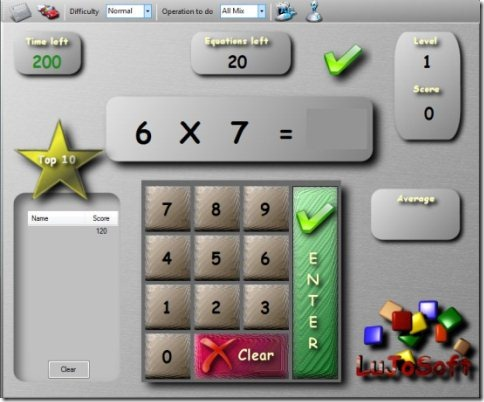Editor Ratings:
User Ratings:
[Total: 1 Average: 1]

Lujosoft KidsMath is a free math game for kids to learn addition, subtraction, multiplication, and division in a fun way. This free math game is specially designed for kids aged between 5 to 9 and offers an easy way to learn math tables starting from 0 to 12.

This math game basically helps you in learning maths by allowing you to solve equations in each level. Each level of this math game includes 20 math equations which your child needs to solve within the displayed time limit.As a user, you have the power to choose the operation and difficulty level. You can choose the operation to do between addition, subtraction, multiplication, and division or mix. Difficulty level can be set between easy, normal, hard and extreme.

So for playing this math game choose your operation, set the difficulty level and click start the game button. The countdown time starts up, so you need to enter the answer for a math equation in the space for answer using number keys or using the number buttons on the game interface. After entering the answer click the enter button for the next equation to come up and to know whether your answer was correct.  After you clear a level, it takes you to the next level and so on.

Also, check out few online math games on Mathchimp.

## Features of this Free Math Game:

• Learn addition, subtraction, multiplication and division, all of them playfully.
• Choose the difficulty level between easy, normal, difficult  and extreme.
• Solve 20 math equations in each level.
• Offer choices of operations among addition, subtraction, multiplication, division or mix.
• Easy to play math game.